Next: Discussion Up: Simulation of STM images Previous: Geometric line cut

# Quantum line cut

We have performed quantum mechanical probability current calculations through a simple two dimensional (2D) jellium potential modeling the STM tip - nanotube - support system. STM constant current feedback loop was simulated by moving the tip along a line (''quantum line cut'' ) where the transferred probability was constant.

The 2D model potential has the value zero outside the effective surfaces of the electrodes and the value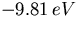inside. (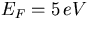,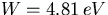).

Tunneling probability is calculated from time dependent scattering of a Gaussian wave packet approaching the model potential from the bulk of the tip. The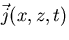probability current density was calculated along a horizontal line inside the support bulk (at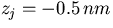). Line integration ofalong this line gives the I(t) probability current and the tunneling probability is obtained as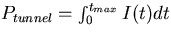.

Probability density of the scattered wave packet is shown on Fig. 6..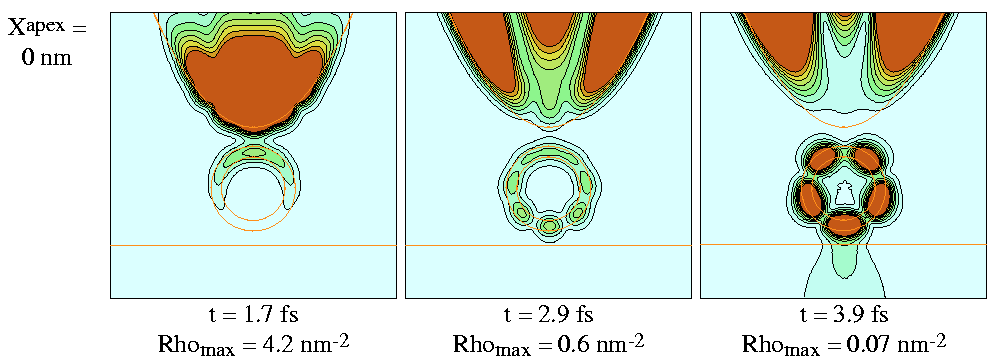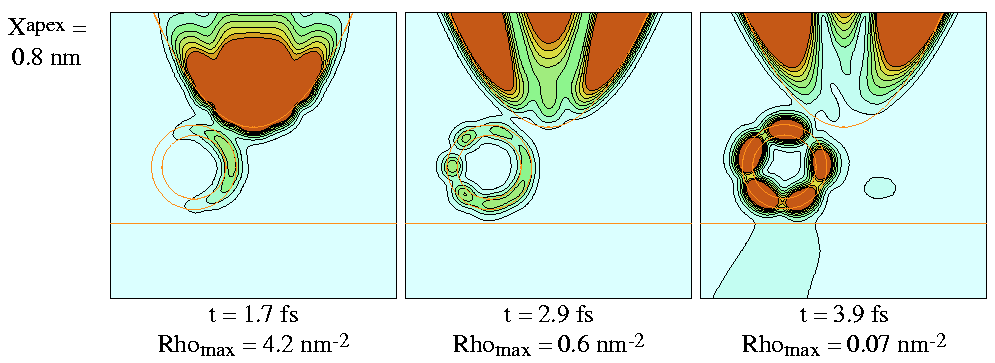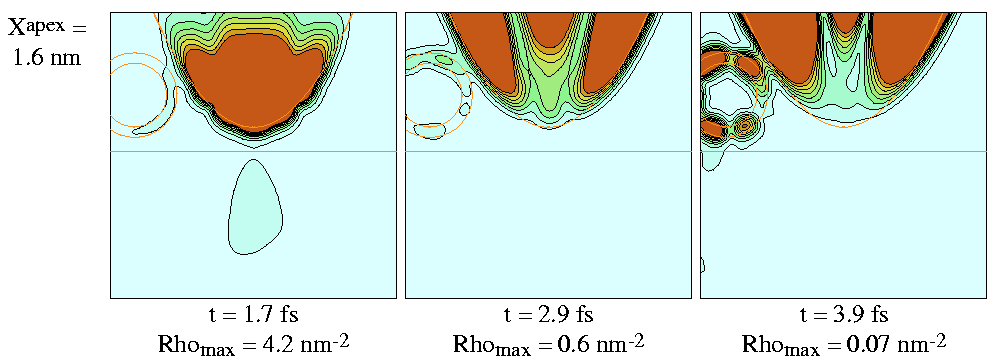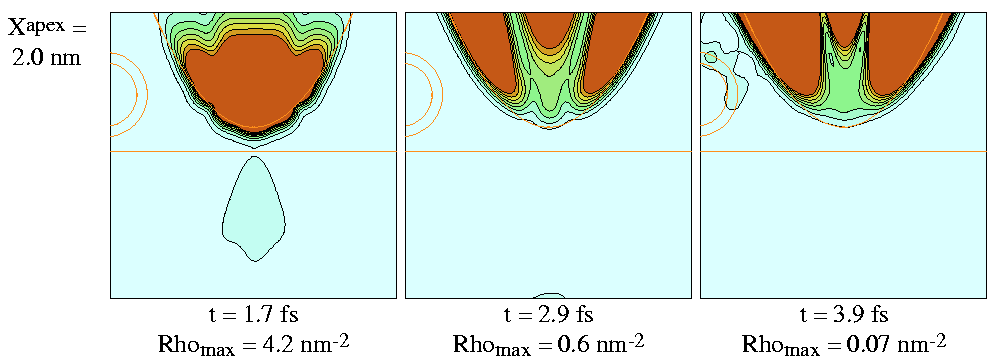Figure 6: Probability density of scattered wave packet for selected time instants and for selected Xapex lateral tip displacements. Effective surfaces of electrodes are shown by red lines. Size of presentation window is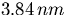. Contour lines are drawn on sqare root scale. Each frame is normalized to its maximum density. Initial momentum of Gaussian wave packet is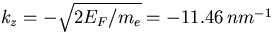and its initial with is.

• For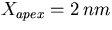the tip is far from the nanotube. Wave packet is tunneling simply from the tip apex into to plane support.
• For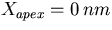tip is above the uppermost point of nanotube. It is a resonant tunneling situation. The probability which remained in the tube region forms standing waves and is leaking into the tip and into the support in distinct impulses.
• For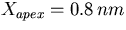(oblique incidence) majority of the probability flows out of the tip at its side around the nearest points of the sample and tip.
• For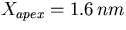we can observe switching of the tunneling points.

 Xapex (nm) Forbidden Tube Total Interface = Forbidden + Tube 0.0 0.071 0.084 0.155 0.8 0.079 0.123 0.202 1.6 0.060 0.020 0.080 2.0 0.057 0.0001 0.057

STM constant current loop was simulated by finding for each fixed Xapex lateral tip displacement that appropriate Zapex vertical tip displacement which yielded a setpoint tunneling probability value of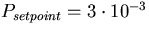. ( Xapex , Zapex ) tip displacement values resulting from this procedure are shown on Fig. 7. by crosses.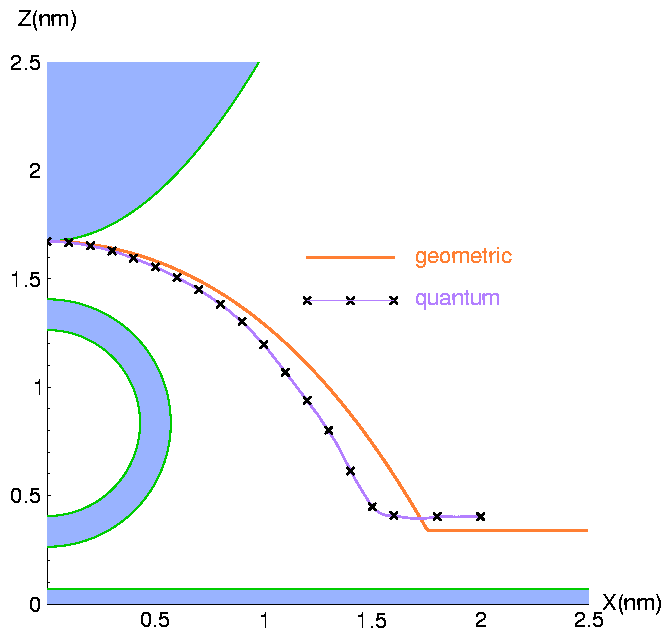Figure 7: Comparison of geometric and quantum line cut. Thick red line is the geometric line cut. Crosses show calculated points of quantum line cut.Next: Discussion Up: Simulation of STM images Previous: Geometric line cut 